Question

Automatic Control IV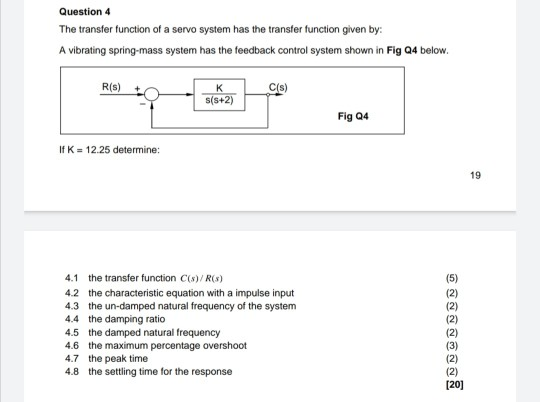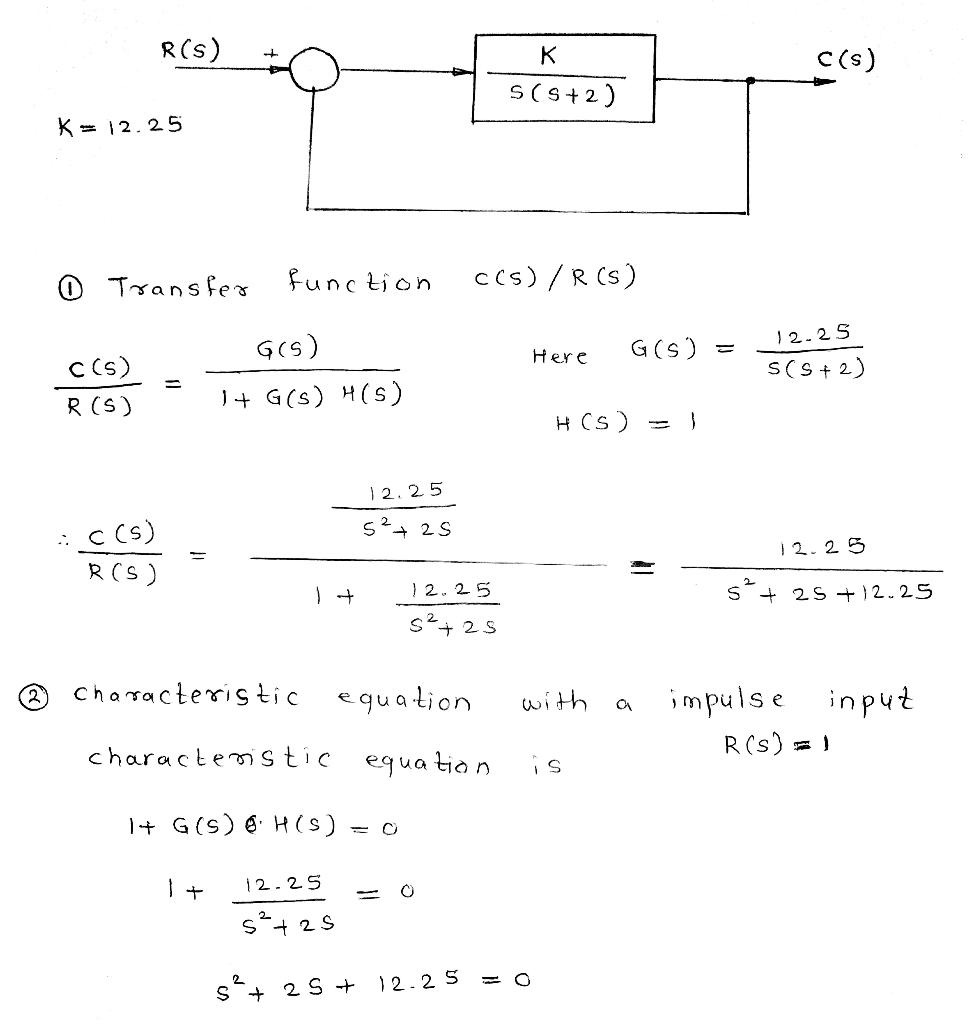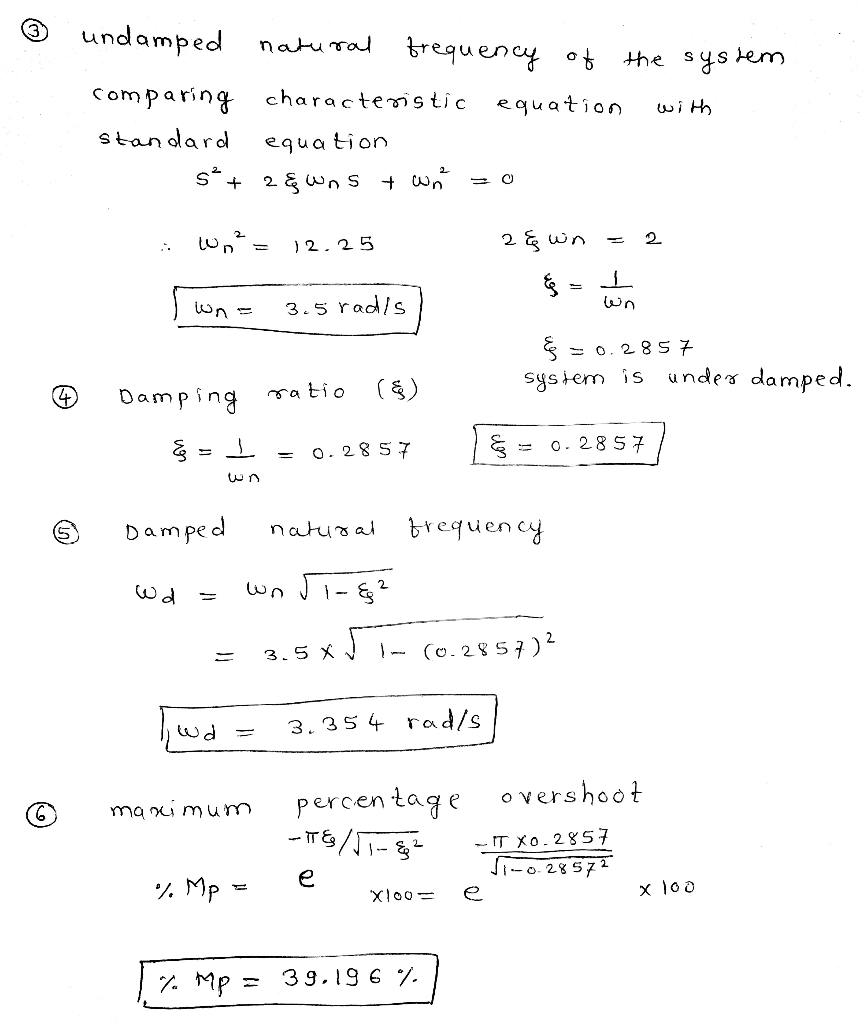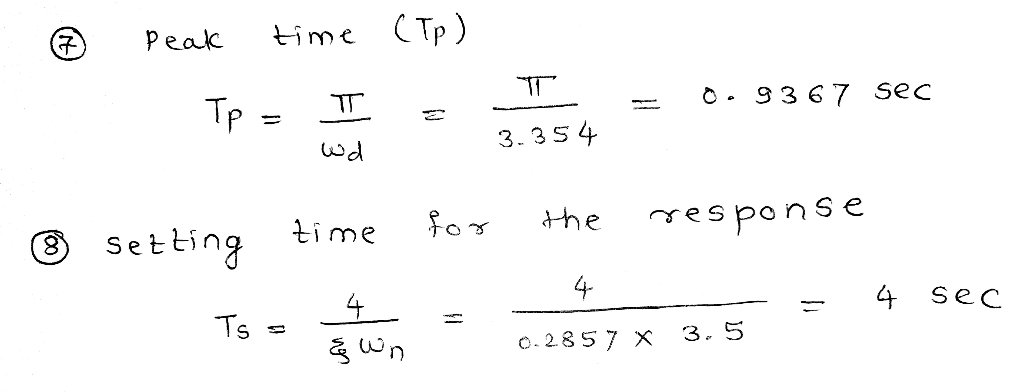#### Earn Coins

Coins can be redeemed for fabulous gifts.

Similar Homework Help Questions
• ### Exercise 3 (15pts) A control system is given by the second order transfer function bellow: Natural frequency of oscillations Damped ratio Determine the range of values of K that render the system...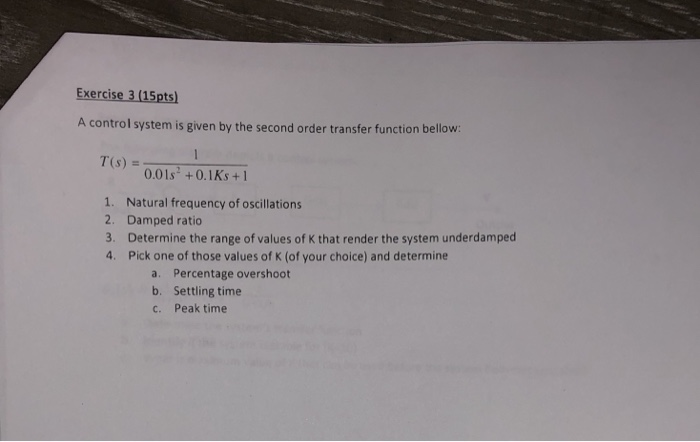Exercise 3 (15pts) A control system is given by the second order transfer function bellow: Natural frequency of oscillations Damped ratio Determine the range of values of K that render the system underdamped Pick one of those values of K (of your choice) and determine 1. 2. 3. 4. a. Percentage overshoot b. Settling time c. Peak time Exercise 3 (15pts) A control system is given by the second order transfer function bellow: Natural frequency of oscillations Damped ratio Determine...

• ### Question three The figure below shows a unit step response of a second order system. From...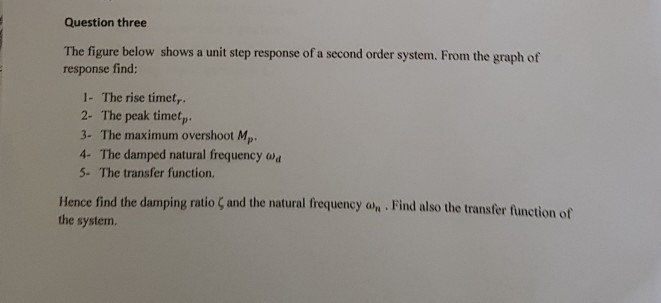Question three The figure below shows a unit step response of a second order system. From the graph of response find: 1- The rise timet, 2- The peak timet, 3- The maximum overshoot Mp 4- The damped natural frequency w 5. The transfer function. Hence find the damping ratio ζ and the natural frequency ah-Find also the transfer function of the system. r 4 02 15 25 35 45 Question Four For the control system shown in the figure below,...

• ### Q3. Consider a single loop unity feedback control system of the open loop transfer function (a) Find the range of values of the gain K and the parameter p so that: (i) The overshoot is less than 10%....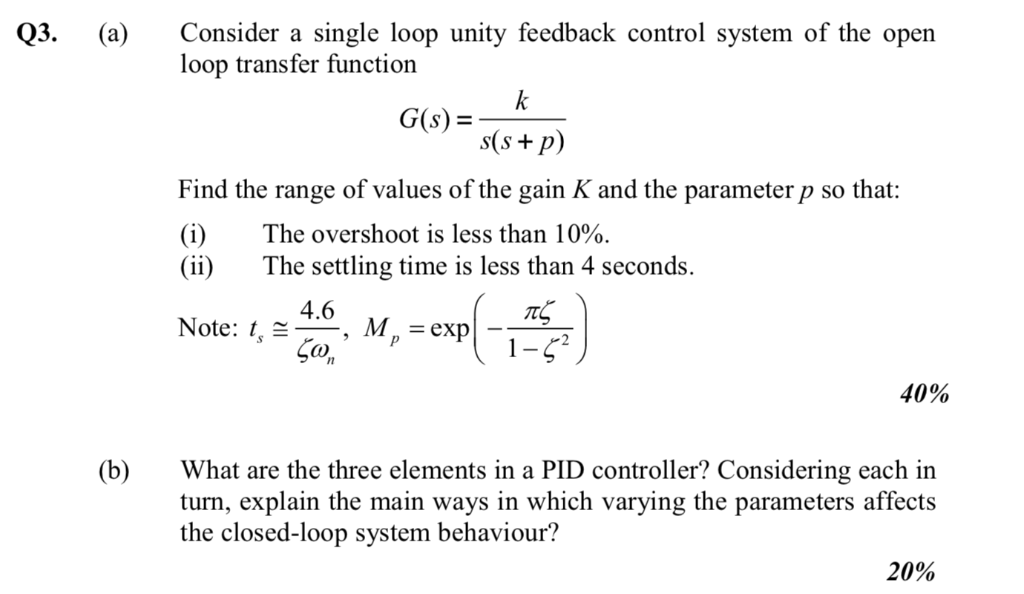Q3. Consider a single loop unity feedback control system of the open loop transfer function (a) Find the range of values of the gain K and the parameter p so that: (i) The overshoot is less than 10%. (ii)The settling time is less than 4 seconds Note: , 4.6 M. = exp CO 40% (b)What are the three elements in a PID controller? Considering each in turn, explain the main ways in which varying the parameters affects the closed-loop system...

• ### Determine: 1. The transfer function C(s)/R(s). Also find the closed-loop poles of the system. 2. The...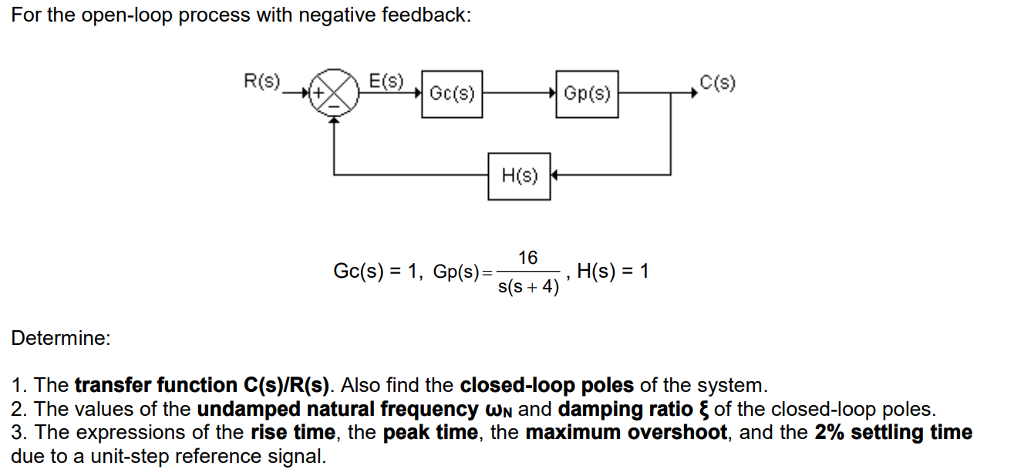Determine: 1. The transfer function C(s)/R(s). Also find the closed-loop poles of the system. 2. The values of the undamped natural frequency ωN and damping ratio ξ of the closed-loop poles. 3. The expressions of the rise time, the peak time, the maximum overshoot, and the 2% settling time due to a unit-step reference signal. For the open-loop process with negative feedback R(S) Gp(S) C(s) H(s) 103 Go(s) = 1 , Gp(s)- s(s + 4) Determine: 1. The transfer function...

• ### Partial Question 5 10/15 pts Given the poles of a transfer function are -2+5j and -2-5j,...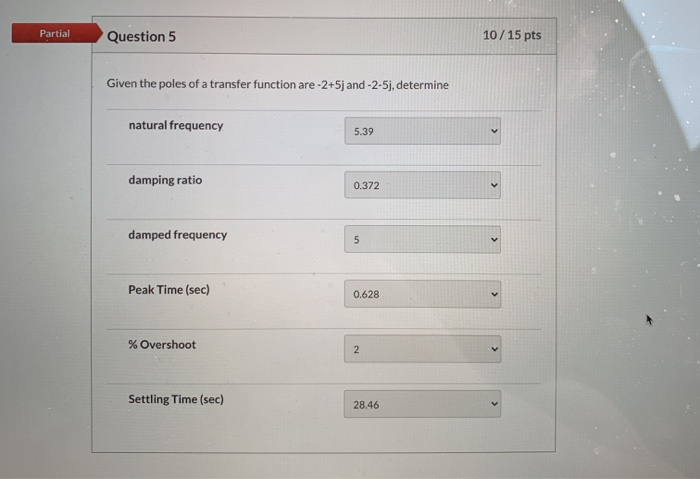Partial Question 5 10/15 pts Given the poles of a transfer function are -2+5j and -2-5j, determine natural frequency 5.39 damping ratio 0.372 damped frequency 5 Peak Time (sec) 0.628 % Overshoot 2 Settling Time (sec) 28.46

• ### 16 The transfer function of a system given by, G(s) = 32 +33 +16' find the...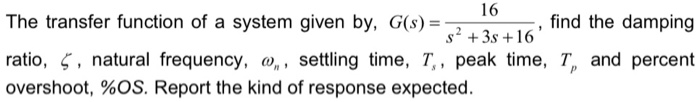16 The transfer function of a system given by, G(s) = 32 +33 +16' find the damping ratio, 5, natural frequency, 0,, settling time, T., peak time, T, and percent overshoot, %OS. Report the kind of response expected. P

• ### Do only parts C and D 1. A second-order system has the following transfer function that...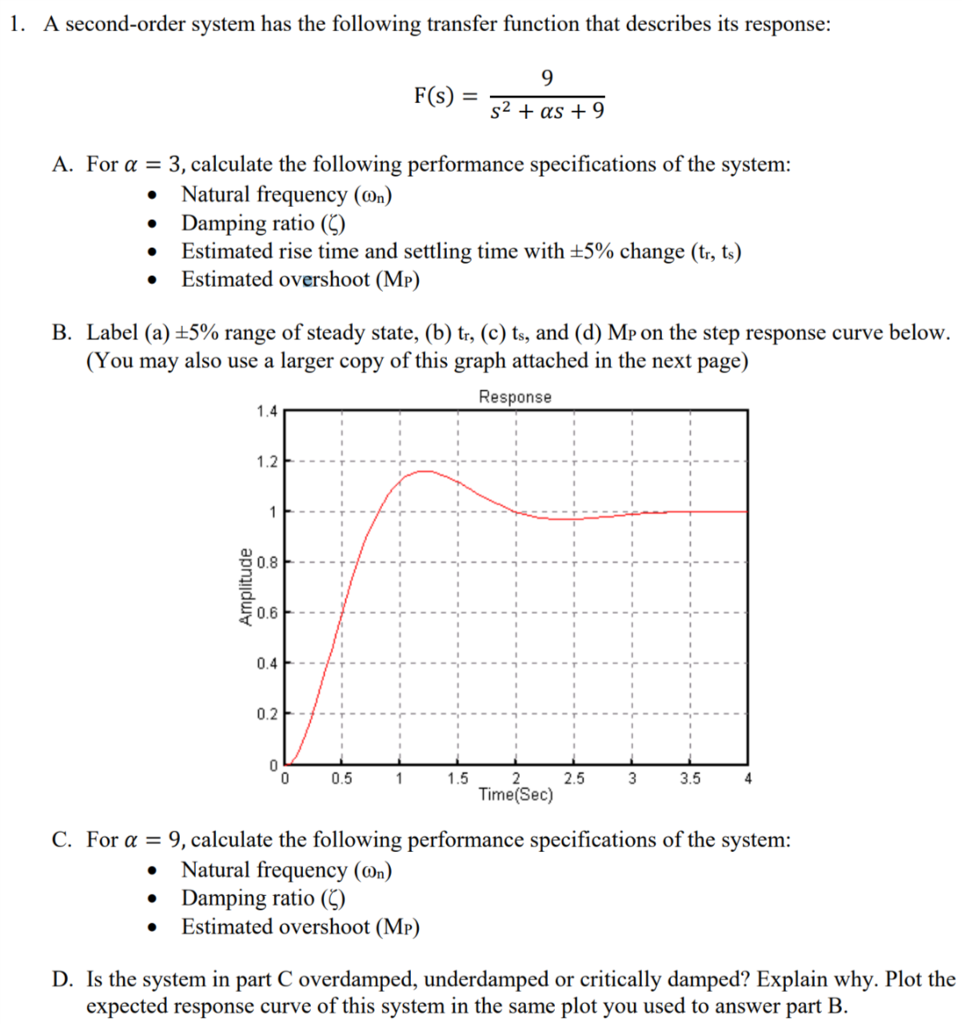Do only parts C and D 1. A second-order system has the following transfer function that describes its response: F(s)- s2 +as + 9 A. For a -3, calculate the following performance specifications of the system: Natural frequency (on) Damping ratio( Estimated rise time and settling time with ±5% change (tr, ts) Estimated overshoot (MP) . B. Label (a) ±5% range of steady state, (b) tr, (c) ts, and (d) MP on the step response curve below (You may also...

• ### 6, Fig. 4 shows three systems. System 1 is a positional servo system. System Π is a positional servo system with PD con...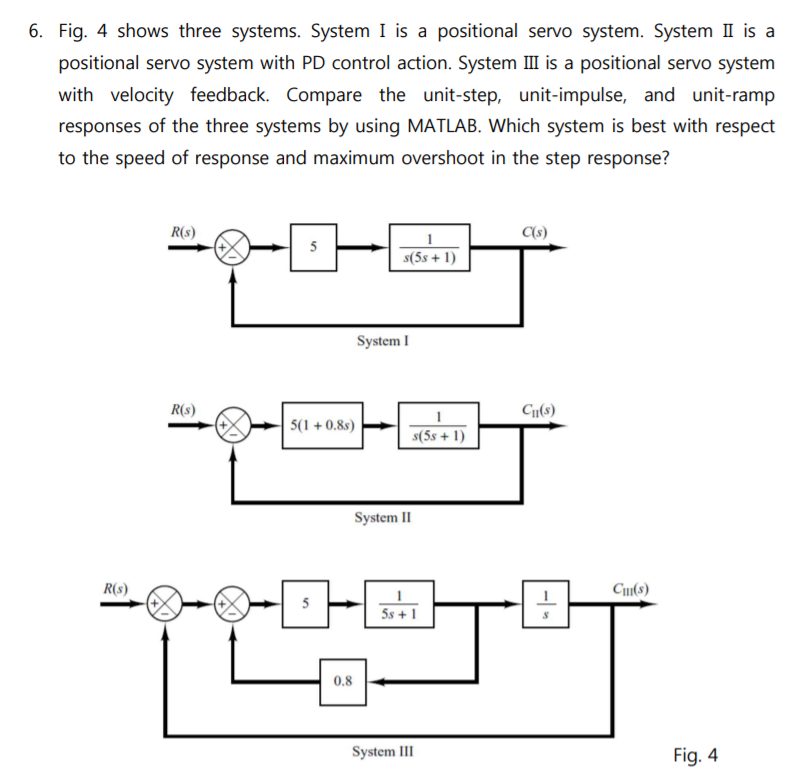6, Fig. 4 shows three systems. System 1 is a positional servo system. System Π is a positional servo system with PD control action. System III is a positional servo system with velocity feedback. Compare the unit-step, unit-impulse, and unit-ramp responses of the three systems by using MATLAB. Which system is best with respect to the speed of response and maximum overshoot in the step response? R(s) C(s) #5s + 1) System I R(s) CI(s S1 +0.8)6-D) System II R(s)...

• ### For the circuit obtain the transfer function E (0) / E (i) and determine the values ​​of the resi...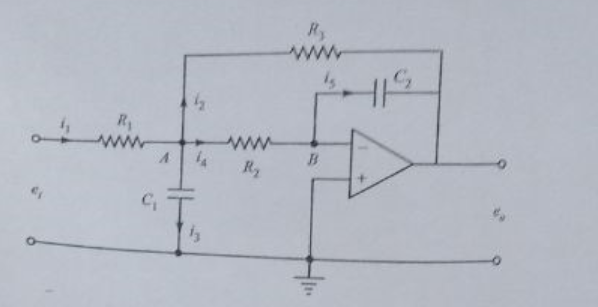For the circuit obtain the transfer function E (0) / E (i) and determine the values ​​of the resistors and capacitors such that the system has a relative damping factor of 0.7 and natural damped frequency of 5rad / sec Draw the entrance and exit in front of the entry and exit time for a unit ramp Find the times ts, tp, tr (for a criterion of 2%) and overshoot of 4% relative damping factor of 0.7 natural damped frequency...

• ### Find the closed time transfer function of the control system as follows: (1) peak time, (2)...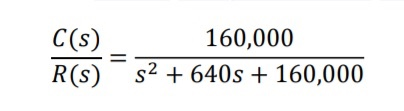Find the closed time transfer function of the control system as follows: (1) peak time, (2) percent overshoot, and (3) settling time. [please show all steps] C(s) R(S) 160,000 52 + 640s + 160,000